1. /
2. CBSE
3. /
4. Class 06
5. /
6. Mathematics
7. /
8. NCERT Solutions for Class...

# NCERT Solutions for Class 6 Maths 3.5

NCERT solutions for Maths Playing with numbers## NCERT Solutions for Class 6 Maths Playing with numbers

###### Question 1.Which of the following statements are true:

(a)If a number is divisible by 3, it must be divisible by 9.

(b)If a number is divisible by 9, it must be divisible by 3.

(c)If a number is divisible by 18, it must be divisible by both 3 and 6.

(d)If a number is divisible by 9 and 10 both, then it must be divisible by 90.

(e)If two numbers are co-primes, at least one of them must be prime.

(f)All numbers which are divisible by 4 must also by divisible by 8.

(g)All numbers which are divisible by 8 must also by divisible by 4.

(h)If a number is exactly divides two numbers separately, it must exactly divide their sum.

(i)If a number is exactly divides the sum of two numbers, it must exactly divide the two numbers separately.

Answer: Statements (b), (c), (d), (g) and (h) are true.

###### Question 2.Here are two different factor trees for 60. Write the missing numbe

(a)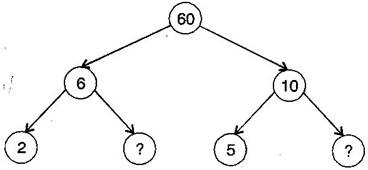(b)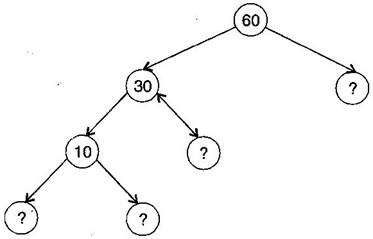(a)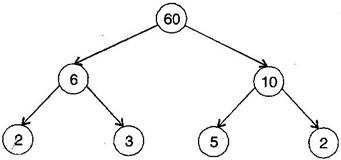(b)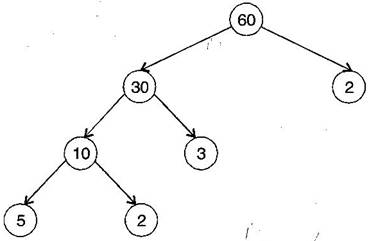NCERT Solutions for Class 6 Maths 3.5

###### Question 4.Write the greatest 4-digit number and express it in terms of its prime facto

Answer: The greatest four digit number is 9999.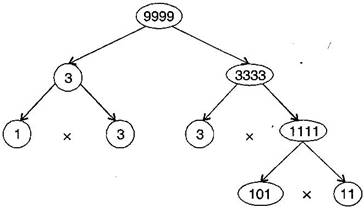The prime factors of 9999 are 3 x 3 x 11 x 101.

NCERT Solutions for Class 6 Maths 3.5

###### Question 5.Write the smallest 5-digit number and express it in terms of its prime facto

Answer: The smallest five digit number is 10000.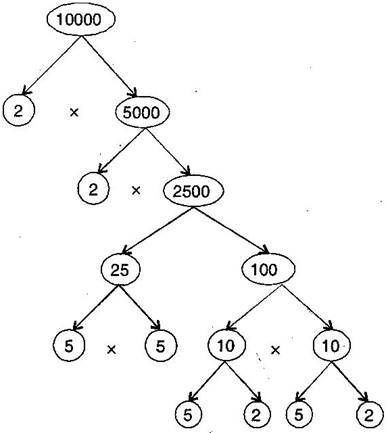The prime factors of 10000 are 2 x 2 x 2 x 2 x 5 x 5 x 5 x 5.

###### Question 6.Find all the prime factors of 1729 and arrange them in ascending order. Now state the relation, if any, between, two consecutive prime numbe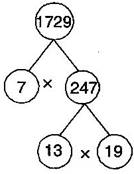Prime factors of 1729 are 7 x 13 x 19.

The difference of two consecutive prime factors is 6.

NCERT Solutions for Class 6 Maths 3.5

###### Question 7.The product of three consecutive numbers is always divisible by 6. Verify this statement with the help of some examples.

Answer: Among the three consecutive numbers, there must be one even number and one multiple of 3. Thus, the product must be multiple of 6.

Example:(i) 2 x 3 x 4 = 24

(ii) 4 x 5 x 6 = 120

###### Question 8.The sum of three consecutive numbers is always divisible by 4. Verify this statement with the help of some examples.

Answer: 3 + 5 = 8 and 8 is divisible by 4.

5 + 7 = 12 and 12 is divisible by 4.

7 + 9 = 16 and 16 is divisible by 4.

9 + 11 = 20 and 20 is divisible by 4.

NCERT Solutions for Class 6 Maths 3.5

###### Question 9.In which of the following expressions, prime factorization has been done:

(a)24 = 2 x 3 x 4

(b)56 = 7 x 2 x 2 x 2

(c)70 = 2 x 5 x 7

(d)54 = 2 x 3 x 9

Answer: In expressions (b) and (c), prime factorization has been done.

###### Question 10.Determine if 25110 is divisible by 45.
[Hint: 5 and 9 are co-prime numbe Test the divisibility of the number by 5 and 9.]

Answer: The prime factorization of 45 = 5 x 9

25110 is divisible by 5 as ‘0’ is at its unit place.

25110 is divisible by 9 as sum of digits is divisible by 9.

Therefore, the number must be divisible by 5 x 9 = 45

NCERT Solutions for Class 6 Maths 3.5

###### Question 11. 18 is divisible by both 2 and 3. It is also divisible by 2 x 3 = 6. Similarly, a number is divisible by 4 and 6. Can we say that the number must be divisible by 4 x 6 = 24? If not, give an example to justify your answer.

Answer: No. Number 12 is divisible by both 6 and 4 but 12 is not divisible by 24.

Question 12.I am the smallest number, having four different prime facto Can you find me?

Answer: 2 x 3 x 5 x 7 = 210

## NCERT Solutions for Class 6 Maths 3.5

NCERT Solutions Class 6 Maths PDF (Download) Free from myCBSEguide app and myCBSEguide website. Ncert solution class 6 Maths includes text book solutions from Class 6 Maths Book . NCERT Solutions for CBSE Class 6 Maths have total 14 chapters. 6 Maths NCERT Solutions in PDF for free Download on our website. Ncert Maths class 6 solutions PDF and Maths ncert class 6 PDF solutions with latest modifications and as per the latest CBSE syllabus are only available in myCBSEguide.

## CBSE app for Students

To download NCERT Solutions for Class 6 Maths, Social Science Computer Science, Home Science, Hindi English, Maths Science do check myCBSEguide app or website. myCBSEguide provides sample papers with solution, test papers for chapter-wise practice, NCERT solutions, NCERT Exemplar solutions, quick revision notes for ready reference, CBSE guess papers and CBSE important question papers. Sample Paper all are made available through the best app for CBSE students and myCBSEguide website.Test Generator

Create question paper PDF and online tests with your own name & logo in minutes.myCBSEguide

Question Bank, Mock Tests, Exam Papers, NCERT Solutions, Sample Papers, Notes

### 4 thoughts on “NCERT Solutions for Class 6 Maths 3.5”

1. It help me to solve my sums and to learn mathematics